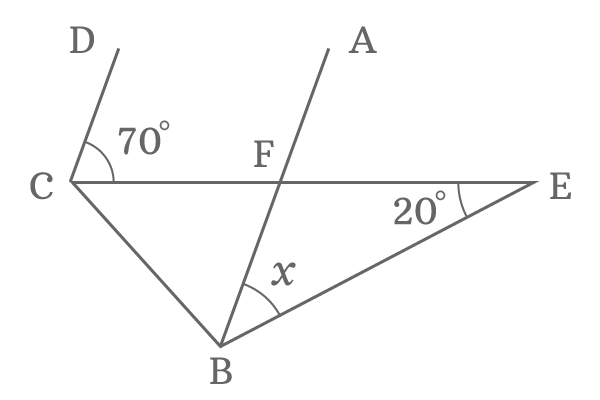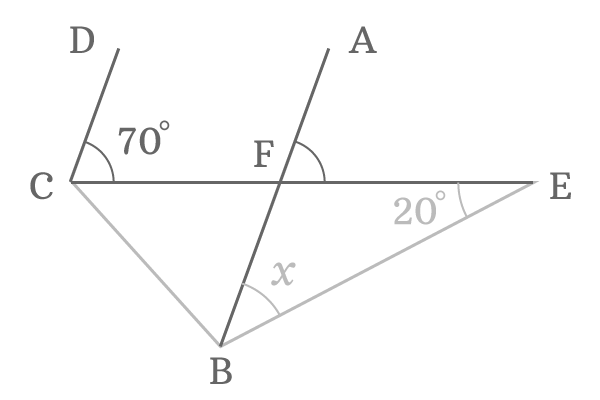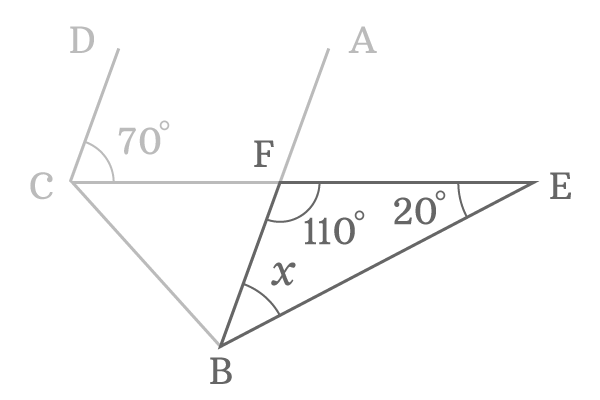# Find $\angle FBE$ if $\angle DCE = 70^\circ$, $\angle FEB = 20^\circ$ and $AB \parallel CD$

$\overline{AB}$ and $\overline{CD}$ are two line segments but they both are parallel lines.The line segment $\overline{CD}$ is intersected the horizontal line $\overline{CE}$ with an angle $70^\circ$. The line segment $\overline{AB}$ is also intersected the same line at point $F$.

The point $C$ is connected to point $B$ by a line segment but the point $E$ is joined to point $B$ with an angle $20^\circ$.

The angle of $\overline{AB}$ with line segment $\overline{BE}$ is unknown and it is an angle that we should have to find in this problem. Assume, $\angle FBE$ is $x$.

###### Step: 1The line segments $\overline{AB}$ and $\overline{CD}$ are parallel lines. Geometrically, the angle made by one line is equal to the angle made by second line.

The angle of the line segment $\overline{AB}$ is $\angle AFE$ and the angle of the line segment $\overline{CD}$ is $\angle DCE$. They both are equal in value due to the parallelism.

$\angle AFE = \angle DCE$

The value of $\angle DCE$ is $70^\circ$. Hence, the $\angle AFE$ is also equal to $70^\circ$.

$\therefore \,\,\,\,\,\, \angle AFE = 70^\circ$

###### Step: 2In $\Delta BEF$, there is one known angle but two unknown angles and it is not possible to find the $\angle FBE$ without knowing the $\angle EFB$. Hence, let us first try to find the value of $\angle EFB$.

The line segment $\overline{AB}$ is a straight line. So, its angle is $180^\circ$ and represented as $\angle AFB$. The line segment $\overline{EF}$ divides the $\angle AFB$ as two angles and they are $\angle AFE$ and $\angle EFB$. So, the sum of them equal to the angle of the line segment $\overline{AB}$.

$\angle AFE + \angle EFB = \angle AFB$

It is determined that the value of $\angle AFE$ is $70^\circ$ in step one and the angle of the line segment $\overline{AB}$ is $180^\circ$. Use this data and find the angle $EFB$.

$70^\circ + \angle EFB = 180^\circ$

$\implies \angle EFB = 180^\circ -70^\circ$

$\therefore \,\,\,\,\,\, \angle EFB = 110^\circ$

###### Step: 3It is derived that the $\angle EFB$ is $110^\circ$ and the $\angle BEF$ is $20^\circ$ in $\Delta EFB$. Now, the third interior angle can easily find by applying the sum of angles of triangle rule.

$\angle FBE + \angle BEF + \angle EFB = 180^\circ$

$\implies x + 20^\circ + 110^\circ = 180^\circ$

$\implies x + 130^\circ = 180^\circ$

$\implies x = 180^\circ -130^\circ$

$\therefore \,\,\,\,\,\, x = 50^\circ$

Therefore, it is derived that the value of $\angle FBE$ is $50^\circ$ and it is the required solution for this geometry problem.

Latest Math Topics
Latest Math Problems

A best free mathematics education website for students, teachers and researchers.

###### Maths Topics

Learn each topic of the mathematics easily with understandable proofs and visual animation graphics.

###### Maths Problems

Learn how to solve the maths problems in different methods with understandable steps.

Learn solutions

###### Subscribe us

You can get the latest updates from us by following to our official page of Math Doubts in one of your favourite social media sites.